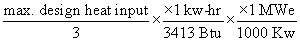# Title 40

## Appendix D to Part 72 - Calculation of Potential Electric Output Capacity

### 40:18.0.1.1.1.11.1.1.4 : Appendix D

Appendix D to Part 72 - Calculation of Potential Electric Output Capacity

The potential electrical output capacity is calculated from the maximum design heat input from the boiler by the following equation:For example:

(1) Assume a boiler with a maximum design heat input capacity of 340 million Btu/hr.

(2) One-third of the maximum design heat input capacity is 113.3 mmBtu/hr. The one-third factor relates to the thermodynamic efficiency of the boiler.

(3) To express this in MWe, the standards conversion of 3413 Btu to 1 kw-hr is used: 113.3 × 10 6 Btu/hr × 1 kw-hr / 3413 Btu × 1 MWe / 1000 kw = 33.2 MWe

[58 FR 15649, Mar. 23, 1993]